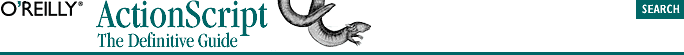home | O'Reilly's CD bookshelfs | FreeBSD | Linux | Cisco | Cisco Exam## 5.5. The Comparison Operators

The interpreter will attempt to convert any nonstring or nonnumeric data value used in a comparison operation to the string or number type. We'll consider the effect of datatype conversions on comparison operations after we discuss the comparison operators themselves.

### 5.5.3. The Less-Than-or-Equal-to Operator

`operand1 <= operand2`

If the operands are numeric, the less-than-or-equal-to operator returns the Boolean true if operand1 is mathematically smaller than or equal to operand2:

```5 <= 6       // true
5 <= 5       // true; note the difference from 5 < 5
-3 <= -6     // false
-6 <= -3     // true```
```"a" <= "z"        // true; lowercase "a" comes before lowercase "z"
"A" <= "a"        // true; although not equal, "A" comes before "a"
"Z" <= "a"        // true; uppercase letters come before lowercase
"hello" <= "hi"   // true; "e" is less than "i"```

Note that the <= operator is written with the equal sign after the less-than sign. The following is not a valid operator: =<.

### 5.5.4. The Greater-Than-or-Equal-to Operator

`operand1 >= operand2`

If the operands are numeric, the greater-than-or-equal-to operator returns the Boolean true if operand1 is mathematically larger than or equal to operand2:

```5 >= 6       // false
5 >= 5       // true; note the difference from 5 > 5
-3 >= -6     // true
-6 >= -3     // false```
```"a" >= "z"        // false; lowercase "a" comes before lowercase "z"
"A" >= "a"        // false; "A" comes before "a" and they are not equal
"Z" >= "a"        // false; uppercase letters come before lowercase
"hello" >= "hi"   // false: "e" is less than "i"```

Note that the >= operator is written with the equal sign after the greater-than sign. The following is not a valid operator: =>.

### 5.5.5. Comparison Operations and Datatype Conversion

1. If both operands are numbers, compare the operands mathematically and return the result. If either number is (or both numbers are) NaN, the result of the comparison is false except in the case of the != operator.

2. If one operand is a number and the other is a string, convert the string to a number and go back to step 1.

3. If either operand is a Boolean, null, or undefined, convert the operand to a number and go back to step 1.

4. If either operand is an object, invoke its valueOf ( ) method to convert the object to a primitive value and go back to step 1. If the valueOf ( ) method fails or does not return a primitive value, return false.

5. Return false.

Note that type conversions performed during a comparison do not alter the original item's stored value or datatype. The results of the temporary conversion are discarded once the expression has been evaluated.

Here is a simple conversion example comparing two Booleans:

`false < true      // true: 0 is less than 1`

Comparison operators always convert composite datatypes to strings or numbers for comparison. In the following example, because both someObj and someOtherObj are members of the Object class, their string value, "[object Object]", is the same:

```someObj = new Object( );
someOtherObj = new Object( );
someObj <= someOtherObj;    // true!```

In the next example, even though "A" has the code point 65, converting "A" to a number yields NaN, which means the whole expression yields false. Use the charCodeAt( ) function to check a string's code point:

```"A" <= 9999                // false
"A".charCodeAt(0) < 9999   // true```